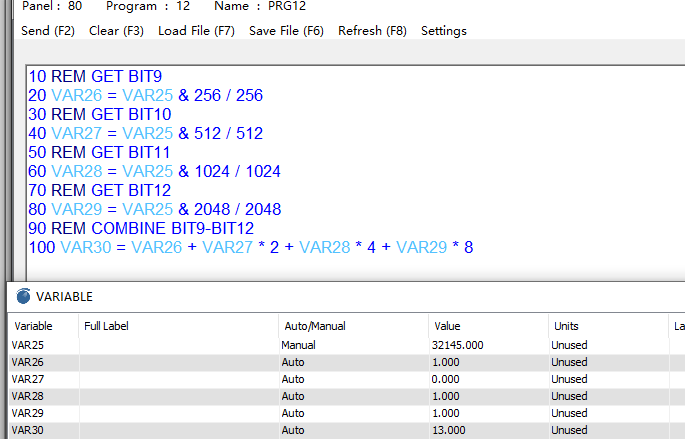“The following are examples of reading a subnet device with Modbus ID=10 connected to a master controller on the Ethernet network with ‘Panel ID’ = 97
10 VAR10 = 97.10.MB_REG7
20 VAR9 = 97.10.MB_REG & 1
30 VAR9 = 97.10.MB_REG | 1
Use & (bitwise and) and | (bitwise or) to manage bit level read & write.”

For example, I have to read data from register 772 and I need bits from 9 to 12. Is it possible?

VAR1 = 1.10MB_REG772

VAR2 = 1.10MB_REG & 9

VAR2 = 1.10MB_REG & 10

VAR2 = 1.10MB_REG & 11

VAR2 = 1.10MB_REG & 12

Something like this?

I didn’t succeed with it and can’t find any information.

Thanx

Chelsea will report back on this shortly.

T3000 only support decimal, so we have to transfer to decimal number
BIT9 → 1 0000 0000 => 256
VAR1 = 1.10.MB_REV772 & 256
BIT10 → 10 0000 0000
VAR2 = 1.10.MB_REG772 & 512
BIT11 → 100 0000 0000
VAR3 = 1.10.MB_REG772 & 1024
BIT12 → 1000 0000 0000
VAR4 = 1.10.MB_REG772 & 2048

Hi @chelsea

Thanx. But how can I read the data from 9th to 12 bits together?

VAR1 = 1.10.MB_REV772 & 256
VAR2 = 1.10.MB_REG772 & 512
VAR3 = 1.10.MB_REG772 & 1024
VAR4 = 1.10.MB_REG772 & 2048

VAR5 = VAR1+VAR2+VAR3+VAR4?

One more question.
Does T3000 supports 32 bits registers?

Thanx a lot

T3000 doesn’t support 32 bit register, you can combine 4 bytes to 1 long.

Thanx @chelsea
How to combine it? Which syntax to use?

Hi, @chelsea

16777215 - bit 24, minus one
65535 - bit 17, minus one
For what we use this bits / this numbers?
Why you didn’t multiple the last VAR?

I got the value 4127 but supposed to be 0-15.Here’s an example, take a number of bits from BIT9 to BIT16 and then combine them to one byte.

1 Like

@chelsea got it. Thanx!

And VAR25 = 1.10.MB_REG2049, right?

I just did a simple demo. You can use 1.10.MB_REG2049 to replace VAR25,

Thanx a lot! @chelsea